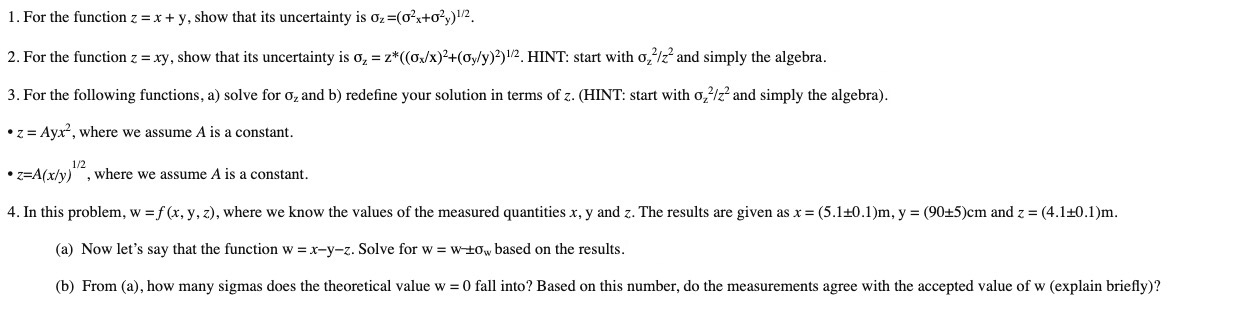# 1. For the function z x + y, show that its uncertainty is oz-(o2+o)122. For the function z = xy, show that its uncertainty is o, z*((o/x )2 + (oyly))1/2. HINT: start with o,/z and simply the algebra3. For the following functions, a) solve for o, and b) redefine your solution in terms of z. (HINT: start with o,/2 and simply the algebra)z Ayx, where we assume A is a constant1/2where we assume A is a constantZEA(x/y)4. In this problem, w f (x, y, z), where we know the values of the measured quantities x, y and z. The results are given as x (5.1+0.1)m, y (9015)cm and z (4.1+0.1)m(a) Now let's say that the function w x-y-z. Solve for w = w+ w based on the results(b) From (a), how many sigmas does the theoretical value w 0 fall into? Based on this number, do the measurements agree with the accepted value of w (explain briefly)?

Question
27 viewshelp_outlineImage Transcriptionclose1. For the function z x + y, show that its uncertainty is oz-(o2+o)12 2. For the function z = xy, show that its uncertainty is o, z*((o/x )2 + (oyly))1/2. HINT: start with o,/z and simply the algebra 3. For the following functions, a) solve for o, and b) redefine your solution in terms of z. (HINT: start with o,/2 and simply the algebra) z Ayx, where we assume A is a constant 1/2 where we assume A is a constant ZEA(x/y) 4. In this problem, w f (x, y, z), where we know the values of the measured quantities x, y and z. The results are given as x (5.1+0.1)m, y (9015)cm and z (4.1+0.1)m (a) Now let's say that the function w x-y-z. Solve for w = w+ w based on the results (b) From (a), how many sigmas does the theoretical value w 0 fall into? Based on this number, do the measurements agree with the accepted value of w (explain briefly)? fullscreen
check_circle

Step 1

As you have posted 4 different questions, we are answering the first question. In case you require the unanswered questions also, kindly re-post them as separate questions.

Step 2

1.

From the given information, the function is z=x+y.

Let us assume that the variables x and y are independent. Hence, Covariance(x, y)=0.

Step 3

Consider...

### Want to see the full answer?

See Solution

#### Want to see this answer and more?

Solutions are written by subject experts who are available 24/7. Questions are typically answered within 1 hour.*

See Solution
*Response times may vary by subject and question.
Tagged in

### Other